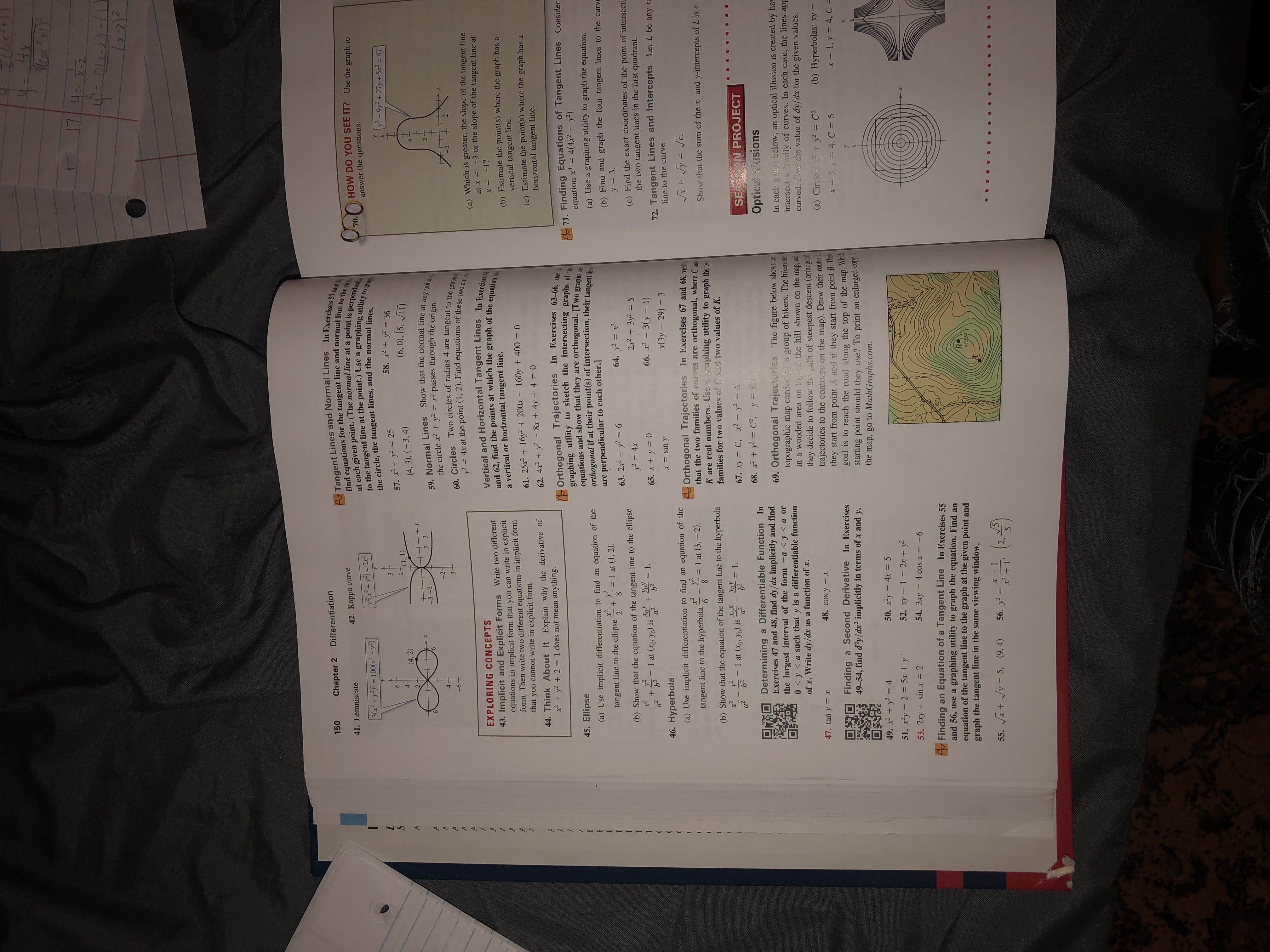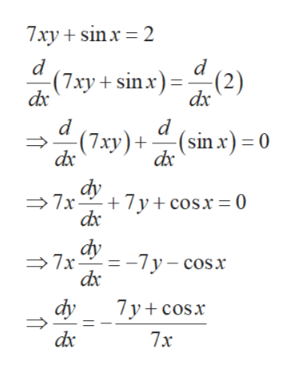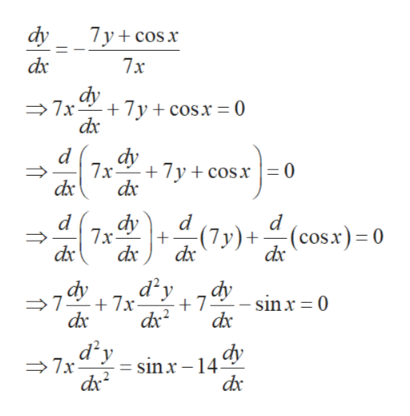# 174oA Tangent Lines and Normal Lines In Exercises 57 and Stfind equations for the tangent line and normal line to the circhat each given point. (The normal line at a point is perpendiculeto the tangent line at the point.) Use a graphing utility to grapDifferentiation150Chapter 242. Каppa curve41. LemniscateHOW DO YOU SEE IT? Use the graph to70.yx2+y2) = 2r?3(2+y2) = 100(2-y)the circle, the tangent lines, and the normal lines.58. x2y2 36(6, 0), (5, VI1)answer the questions.y3-9y2 + 27y+5x? = 47357. x2+y2 = 25(4, 3), (-3, 4)62T(1,1)(4, 2)259. Normal Lines Show that the normal line at any point othe circle x2 +y2 = r2 passes through the origin60. Circles Two circles of radius 4 are tangent to the graph ofy2 = 4x at the point (1, 2). Find equations of these two circleVertical and Horizontal Tangent Lines In Exercises 61and 62, find the points at which the graph of the equation hasx2 3-2-3t2(a) Which is greater, the slope of the tangent lineat x =-3 or the slope of the tangent line atEXPLORING CONCEPTS43. Implicit and Explicit Forms Write two differentequations in implicit form that you can write in explicitform. Then write two different equations in implicit formthat you cannot write in explicit form.Ax=I1?A(b) Estimate the point(s) where the graph has avertical tangent line.a vertical or horizontal tangent line.AA61.25x2 16y2 + 200x 160y +400 = 0(c) Estimate the point(s) where the graph has ahorizontal tangent line.044. Think About It Explain why the derivative ofx2 y22= 1 does not mean62. 4x2+y2- 8x +4y +4anything.AOrthogonal Trajectories In Exercises 63-66graphing utility to sketch the intersecting graphs of theequations and show that they are orthogonal. [Two graphs areorthogonal if at their point(s) of intersection, their tangentperpendicular to each other.]71. Finding Equations of Tangent Lines Considerequation x4 4(4x2 - y2).(a) Use a graphing utility to graph the equation.45. Ellipse(a) Use implicit differentiation to find an equation of theare(b) Find and graph the four tangent lines to the curvey = 3.(c) Find the exact coordinates of the point of intersectithe two tangent lines in the first quadrant.= 1 at (1, 2).8tangent line to the ellipse264. y2 x363. 2x2+y2 6(b) Show that the equation of the tangent line to the ellipse2x2 +3y2 5y2 4xx2 y2+УoУXx(Yo Yo) isa2= 1.66. x3 = 3(y - 1)= 1 at65. x + y = 0a2b272. Tangent Lines and Intercepts Let L be any tax(3y 29) 3= sin y46. Hyperbolaline to the curve(a) Use implicit differentiation to find an equation of theAOrthogonal Trajectories In Exercises 67 and 68, veritthat the two families of crves are orthogonal, where C andK are real numbers. Use raphing utility to graph the twofamilies for two values ofsod two values of K.1 at (3, -2).tangent line to the hyperbola6Show that the sum of the x- and y-intercepts of L is c.(b) Show that the equation of the tangent line to the hyperbolax2y2a2y) isoXYoya2b21 at (x1.67. xy = C, x2- y2 -68. x2 +y2 = C2, y= Eb2SETH N PROJECTDetermining a Differentiable Function InExercises 47 and 48, find dy/dx implicitly and findthe largest interval of the form -a

Question
9 views

53help_outlineImage Transcriptionclose17 4o A Tangent Lines and Normal Lines In Exercises 57 and St find equations for the tangent line and normal line to the circh at each given point. (The normal line at a point is perpendicule to the tangent line at the point.) Use a graphing utility to grap Differentiation 150 Chapter 2 42. Каppa curve 41. Lemniscate HOW DO YOU SEE IT? Use the graph to 70. yx2+y2) = 2r? 3(2+y2) = 100(2-y) the circle, the tangent lines, and the normal lines. 58. x2y2 36 (6, 0), (5, VI1) answer the questions. y3-9y2 + 27y+5x? = 47 3 57. x2+y2 = 25 (4, 3), (-3, 4) 6 2T(1,1) (4, 2) 2 59. Normal Lines Show that the normal line at any point o the circle x2 +y2 = r2 passes through the origin 60. Circles Two circles of radius 4 are tangent to the graph of y2 = 4x at the point (1, 2). Find equations of these two circle Vertical and Horizontal Tangent Lines In Exercises 61 and 62, find the points at which the graph of the equation has x 2 3 -2 -3t 2 (a) Which is greater, the slope of the tangent line at x =-3 or the slope of the tangent line at EXPLORING CONCEPTS 43. Implicit and Explicit Forms Write two different equations in implicit form that you can write in explicit form. Then write two different equations in implicit form that you cannot write in explicit form. A x=I1? A (b) Estimate the point(s) where the graph has a vertical tangent line. a vertical or horizontal tangent line. A A 61.25x2 16y2 + 200x 160y +400 = 0 (c) Estimate the point(s) where the graph has a horizontal tangent line. 0 44. Think About It Explain why the derivative of x2 y22= 1 does not mean 62. 4x2+y2- 8x +4y +4 anything. AOrthogonal Trajectories In Exercises 63-66 graphing utility to sketch the intersecting graphs of the equations and show that they are orthogonal. [Two graphs are orthogonal if at their point(s) of intersection, their tangent perpendicular to each other.] 71. Finding Equations of Tangent Lines Consider equation x4 4(4x2 - y2). (a) Use a graphing utility to graph the equation. 45. Ellipse (a) Use implicit differentiation to find an equation of the are (b) Find and graph the four tangent lines to the curve y = 3. (c) Find the exact coordinates of the point of intersecti the two tangent lines in the first quadrant. = 1 at (1, 2). 8 tangent line to the ellipse 2 64. y2 x3 63. 2x2+y2 6 (b) Show that the equation of the tangent line to the ellipse 2x2 +3y2 5 y2 4x x2 y2 + УoУ Xx (Yo Yo) is a2 = 1. 66. x3 = 3(y - 1) = 1 at 65. x + y = 0 a2 b2 72. Tangent Lines and Intercepts Let L be any ta x(3y 29) 3 = sin y 46. Hyperbola line to the curve (a) Use implicit differentiation to find an equation of the AOrthogonal Trajectories In Exercises 67 and 68, verit that the two families of crves are orthogonal, where C and K are real numbers. Use raphing utility to graph the two families for two values ofsod two values of K. 1 at (3, -2). tangent line to the hyperbola 6 Show that the sum of the x- and y-intercepts of L is c. (b) Show that the equation of the tangent line to the hyperbola x2y2 a2 y) isoXYoy a2 b2 1 at (x 1. 67. xy = C, x2- y2 - 68. x2 +y2 = C2, y= E b2 SETH N PROJECT Determining a Differentiable Function In Exercises 47 and 48, find dy/dx implicitly and find the largest interval of the form -a
check_circle

Step 1

Obtain the first derivative implicitly as follows.help_outlineImage Transcriptionclose7xy +sinx 2 d (7ay + sinx)(2) dx d d (7xy)( (sin x) = 0 dx dy 7x + 7y+cosx=0 dy =-7y-cosr 7x dy 7y+cosx dx 7x fullscreen
Step 2

Compute the second derivative implicitly in terms...help_outlineImage Transcriptionclosedy 7y cos x dx 7x dy 7x 7 cosx = 0 d dy 7cosx = 0 7x dy 7x dx d d (cos x) 0 -(7y)+ dx dx d2y sinx= 0 dy +7x 7 dc 7xysinx-14 fullscreen

### Want to see the full answer?

See Solution

#### Want to see this answer and more?

Solutions are written by subject experts who are available 24/7. Questions are typically answered within 1 hour.*

See Solution
*Response times may vary by subject and question.
Tagged in

### Derivative